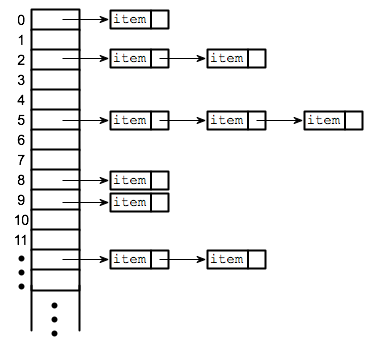# 哈希算法原理【Java实现】

## 散列

hash = hashfunc（key）
index = hash％array_size

        String obj1 = String.valueOf(4);
String obj2 = String.valueOf(16);
String obj3 = String.valueOf(68);
String obj4 = String.valueOf(125);
String obj5 = String.valueOf(255);

System.out.println(obj1.hashCode() % 5);
System.out.println(obj2.hashCode() % 5);
System.out.println(obj3.hashCode() % 5);
System.out.println(obj4.hashCode() % 5);
System.out.println(obj5.hashCode() % 5);### 散列冲突之开放寻址法

index = index % hashTableSize
index = (index + 1) % hashTableSize
index = (index + 2) % hashTableSize
index = (index + 3) % hashTableSize

.......

public class HashTable {

//数组容量
private int capacity;

//哈希键值对数组
private Entry[] entries = {};

public HashTable(int capacity) {
this.capacity = capacity;
entries = new Entry[this.capacity];
}

//添加键值对
public void put(String key, String value) {
final Entry hashEntry = new Entry(key, value);
int hash = getHash(key);
entries[hash] = hashEntry;
}

//获取键哈希值
private int getHash(String key) {
int hashCode = key.hashCode();
int hash = hashCode % capacity;
while (entries[hash] != null) {
hashCode += 1;
hash = hashCode % capacity;
}
return hash;
}

//获取指定键值
public String get(String key) {
int hashCode = key.hashCode();
int hash = hashCode % capacity;
if (entries[hash] != null) {
while (!entries[hash].key.equals(key))
{
hashCode += 1;
hash = hashCode % capacity;
}
return entries[hash].value;
}
return null;
}

private class Entry {
String key;
String value;

public Entry(String key, String value) {
this.key = key;
this.value = value;
}
}
}

public class Main {

public static void main(String[] args) {

HashTable table = new HashTable(5);

table.put(String.valueOf(4), String.valueOf(4));
table.put(String.valueOf(16), String.valueOf(16));
table.put(String.valueOf(68), String.valueOf(68));
table.put(String.valueOf(125), String.valueOf(125));
table.put(String.valueOf(255), String.valueOf(255));

System.out.println(table.get(String.valueOf(4)));
System.out.println(table.get(String.valueOf(125)));
}
}### 散列冲突之链地址法public class HashTable {

//数组容量
private int capacity;

//哈希键值对数组
private Entry[] entries = {};

public HashTable(int capacity) {
this.capacity = capacity;
entries = new Entry[this.capacity];
}

//添加键值对
public void put(String key, String value) {
//获取键哈希值
int hash = getHash(key);

//实例化类存放键和值
final Entry hashEntry = new Entry(key, value);

//如果在数组中未有冲突的键则直接存放
if(entries[hash] == null) {
entries[hash] = hashEntry;
}

//如果找到冲突的哈希值则存放到单链表中的下一引用
else {
Entry temp = entries[hash];
while(temp.next != null) {
temp = temp.next;
}
temp.next = hashEntry;
}
}

//获取键哈希值
private int getHash(String key) {
return key.hashCode() % capacity;
}

//获取指定键值
public String get(String key) {

int hash = getHash(key);

if(entries[hash] != null) {

Entry temp = entries[hash];

while( !temp.key.equals(key)
&& temp.next != null ) {
temp = temp.next;
}
return temp.value;
}

return null;
}

private class Entry {
String key;
String value;
Entry next;

public Entry(String key, String value) {
this.key = key;
this.value = value;
this.next = null;
}
}
}

## 总结

posted @ 2019-09-17 00:04  Jeffcky  阅读(2544)  评论(1编辑  收藏  举报